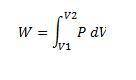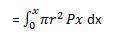# Gas expands in a cylinder

• Lamoid
In summary, when gas expands in a cylinder with radius r, the pressure at any given time is a function of the volume: P = P (V). The force exerted by the gas on the piston is the product of the pressure and the area: F = π(r^2)P. To find the work done by the gas when the volume expands from V1 to V2, we can use the expression W = \int F dx and make the substitution F = P * pi * r^2. Then, we can use the change-of-variable method to change the limits of integration from x1 and x2 to V1 and V2, giving us the final expression of W = \int^{V2}_{V

## Homework Statement

When gas expands in a cylinder with radius r, the pressure at any given time is a function of the volume: P = P (V). The force exerted by the gas on the piston is the product of the pressure and the area: F = π(r^2)P. Show that the work done by the gas when the volume expands from Volume V1 to Volume V2 is

## Homework Equations## The Attempt at a Solution

I was having a problem doing this question so I just sort of trudged ahead to see if I could get something and I ended up using substitution with u = n(r^2)x and du = nr^2 and then adjusted the limits of integration which were x+a and x. I am fairly certain this is wrong though and would appreciate help.

What is the usual definition of work?

Integral of Force times distance

Last edited:
Yes.

Can you make substitutions and rearrange terms to get that integral in terms of P and V?Ok so that's the integral. So I replace (pi)(r^2)x with V ?

Last edited:
Um, wait a moment.

If a force F moves an object over a distance dx, then the work done in that small distance is F*dx.

For extended distances, we would integrate F * dx

Try working with that expression ... you were on the right track otherwise.

Hmm, so I am confused. This is my best guess.

f * dx = W

πr^2P * dx = W

PV = W

P*dv = W'

Then you integrate it using the change in volume as the limits of integration?

Try this:

$$W = \int F dx$$

and do the same substitution F = P * pi * r^2

Okay, when I do that I end up, obviously, W = integral P * pi * r^2 * dx. I know I have to get V's in here, but I am unsure if I substitute pi * r^2 * dx with a V or a dv since I am talking about the change in x.

Lamoid said:
Okay, when I do that I end up, obviously, W = integral P * pi * r^2 * dx. I know I have to get V's in here, but I am unsure if I substitute pi * r^2 * dx with a V or a dv since I am talking about the change in x.

V = $\pi r^2 x$ where we take the origin to be wherever the piston starts. What's dV?

So dv is pi*r^2*dx. Now, must I do anything to make the limits of intergration V1 to V2?

This is a change-of-variable as taught in integral calculus.

With the substitution V = pi r^2 x, the limits simply change accordingly so that:
x1 becomes V1, where V1 = pi r^2 x1
and similarly for x2 and V2.

In other words,

$$\int^{x2}_{x1} ... \ dx$$

becomes

$$\int^{\pi r^2 x2}_{\pi r^2 x1} ... \ dV$$

or just

$$\int^{V2}_{V1} ... \ dV$$

Last edited: